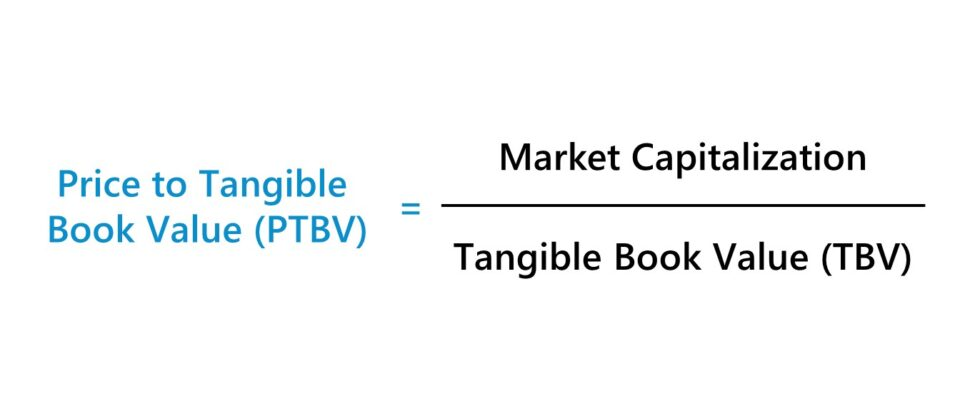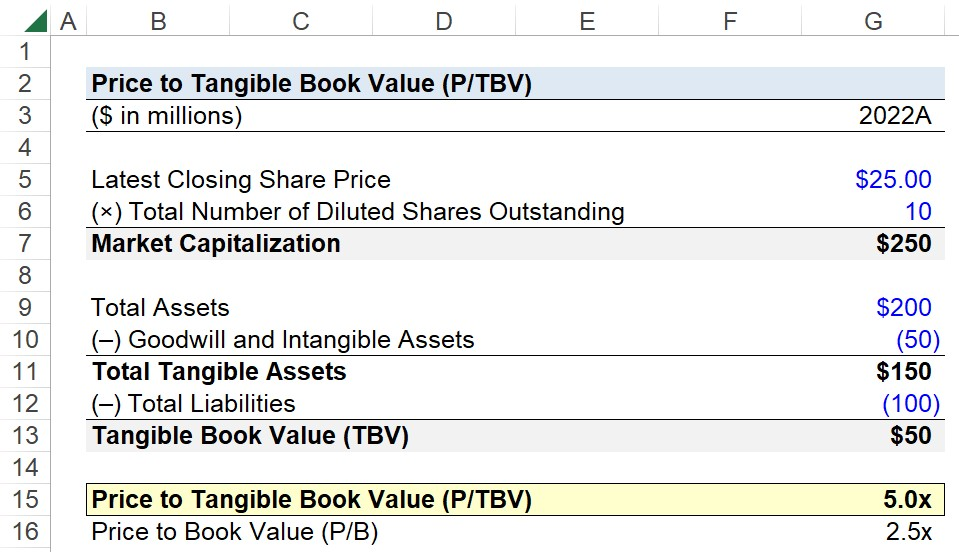Welcome to Wall Street Prep! Use code at checkout for 15% off.# Price to Tangible Book Value (P/TBV)

Guide to Understanding the Price to Tangible Book Value Ratio (P/TBV)## How to Calculate Price to Tangible Book Value?

The price to tangible book value, or P/TBV, is an equity valuation ratio that compares a company’s market value of equity to its tangible book value (TBV).

• Market Capitalization → The market capitalization, or “equity value”, of a company is the total value of its common shares outstanding to its equity holders. In short, the market cap is the fair value of a company’s common equity, i.e. the market value of equity, which is set by the latest transactions in the public markets, as of the present date.
• Tangible Book Value (TBV) → The tangible book value (TBV) measures how much a company’s tangible assets are worth, net of intangible assets, which refer to non-physical assets (e.g. goodwill, intellectual property, trademarks, copyright, patents). Contrary to the market cap, the tangible book value (TBV) is an adjusted accounting metric, where the recorded value of a company’s intangible assets is deducted from the book value of equity (BVE).

Since the tangible book value (TBV) metric removes the value of intangible assets, i.e. non-physical assets, the TBV is a closer representation of the remaining net value belonging to common equity holders post-liquidation, i.e. once all outstanding liabilities on the balance sheet, such as debt, are repaid in full.

For example, the recognition of goodwill on a company’s balance sheet is a required accrual accounting convention intended to capture the excess purchase price paid in excess of the acquired asset’s fair market value (FMV).

Therefore, the tangible book value (TBV) is a more conservative approximation of the value of a post-liquidation company because all outstanding debt and liabilities like accounts payable (A/P) are of higher priority relative to claims held by common shareholders.

The drawback, however, is that TBV is still a rough estimation of a company’s hypothetical liquidation value, as certain intangible assets can in fact possess value and be sold in a liquidation proceeding. In addition, the liquidation value of tangible assets is rarely ever actually equivalent to the value as recorded on the balance sheet.

## Price to Tangible Book Value Formula (P/TBV)

The formula used to calculate the price to tangible book value ratio (P/TBV) is as follows.

Price to Tangible Book Value (P/TBV) = Market Capitalization ÷ Tangible Book Value (TBV)
• Market Capitalization = Latest Closing Share Price × Total Number of Diluted Shares Outstanding
• Tangible Book Value (TBV) = (Total Assets – Intangible Assets) – Total Liabilities

Alternatively, the price to tangible book value ratio (P/TBV) can also be expressed on a per-share basis.

Price to Tangible Book Value (P/TBV) = Market Share Price ÷ Tangible Book Value Per Share (TBVPS)
• Market Share Price = Market Capitalization ÷ Total Number of Diluted Shares Outstanding
• Tangible Book Value Per Share (TBVPS) = Tangible Book Value (TBV) ÷ Total Number of Diluted Shares Outstanding

## Price to Book Ratio (P/B) vs. Price to Tangible Book Value (P/TBV)

The price to book (P/B) and price to tangible book value (P/TBV) are near-identical valuation ratios that compare a market value metric to a bookkeeping metric.

• Price to Book (P/B Ratio) → The P/B ratio compares a company’s market value of equity (i.e. market capitalization) relative to its book value of equity (BVE). The book value of equity (BVE) is equal to a company’s total assets minus its total liabilities, so the metric is inclusive of intangible assets.
• Price to Tangible Book Value (P/TBV) → The P/TBV is virtually identical to the P/B ratio, aside from the additional step of removing the value of intangible assets. For companies with significant amounts of intangible assets recorded on their balance sheets, the P/BV can be distorted and potentially misleading, which is where a more conservative measure like the P/TBV ratio can be more appropriate.

The formula used to calculate the price to book ratio (P/B) is as follows.

Price to Book Ratio (P/B) = Market Capitalization ÷ Book Value of Equity (BVE)

Like the price to book ratio, a lower price to tangible book value ratio is interpreted as a positive sign that the underlying company could potentially be undervalued (or vice versa for higher ratios).

• Lower P/TBV Ratio → Potentially Undervalued Market Pricing
• Higher P/TBV Ratio → Potentially Overvalued Market Pricing

## Price to Tangible Book Value Calculator (P/TBV)

We’ll now move on to a modeling exercise, which you can access by filling out the form below.Submitting...

## 1. Market Value and Balance Sheet Assumptions

Suppose you’re tasked with calculating the price to tangible book value of a company given the following market value and balance sheet assumptions.

Market Value Assumptions

• Latest Closing Share Price = \$25.00
• Total Number of Diluted Shares Outstanding = 10 million

Balance Sheet Financial Assumptions

• Total Assets = \$200 million
• Goodwill and Intangible Assets = \$50 million
• Total Liabilities = \$100 million

## 2. Price to Tangible Book Value Calculation Example

Our first step is to determine our company’s market capitalization, which is the product of its share price as of the latest trading date and its total share count on a diluted basis.

Upon multiplying the company’s most recent share price by the number of its total diluted shares outstanding, we arrive at a market cap of \$250 million.

• Market Capitalization = \$25.00 × 10 million = \$250 million

In the next step, we’ll calculate our company’s tangible book value (TBV), starting with the deduction of goodwill and intangible assets from total assets.

• Total Tangible Assets = \$200 million – \$50 million = \$150 million

From there, the next step is to net the company’s total tangible assets against its total liabilities, which returns a tangible book value (TBV) of \$50 million.

• Tangible Book Value (TBV) = \$150 million – \$100 million = \$50 million

After dividing our company’s market capitalization by its tangible book value (TBV), the implied price to tangible book value ratio (P/TBV) is 5.0x.

• Price to Tangible Book Value (P/TBV) = \$250 million ÷ \$50 million = 5.0x

## 3. P/TBV Ratio Analysis Example

In the final section of our exercise, we’ll compare the P/TBV ratio we calculated in the prior step to the price to book ratio (P/B) using the same assumptions.

The numerator, the market capitalization, is still \$250 million, but the denominator is not adjusted for intangible assets in this case.

Instead, we’ll subtract the company’s total liabilities from the company’s total assets to calculate the book value of equity (BVE) as \$100 million.

• Book Value of Equity (BVE) = \$200 million – \$100 million = \$100 million

With our two inputs determined, the only step left is to divide the market cap by the book value to arrive at a price to book ratio (P/B) of 2.5x.

• Price to Book (P/B Ratio) = \$250 million ÷ \$100 million = 2.5x

In comparison to the price to book ratio (P/B), the price to tangible book value ratio (P/TBV) is double that value, which reflects how the P/TBV can be a more practical tool to better understand the current market valuation of a company in certain circumstances.Step-by-Step Online Course

### Everything You Need To Master Financial Modeling

Enroll in The Premium Package: Learn Financial Statement Modeling, DCF, M&A, LBO and Comps. The same training program used at top investment banks.